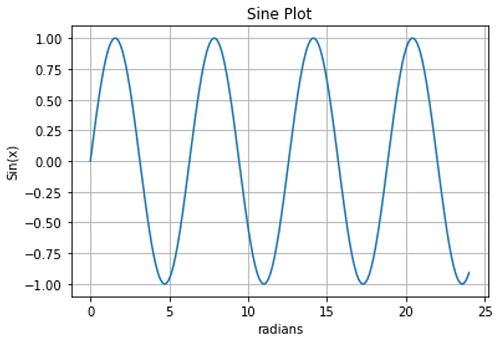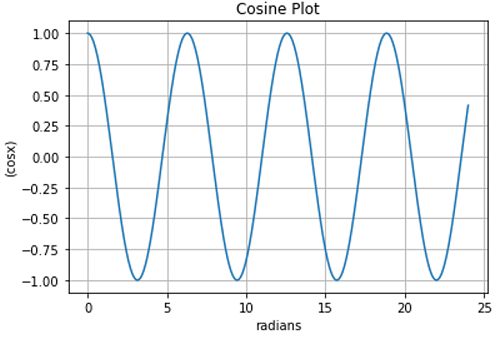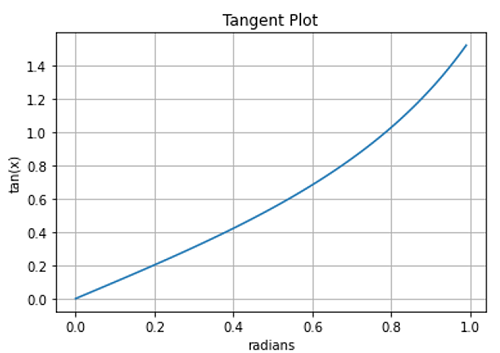# Python | Plotting Trigonometric Functions

In this article, we are going to learn about the various plotting trigonometric functions and their Python implementations.
Submitted by Anuj Singh, on July 15, 2020

Trigonometry is one of the most important parts in engineering and many times, therefore matplotlib.pyplot in combination with NumPy can help us to plot our desired trigonometric functions. In this article, we are going to introduce a few examples of trig-functions.

## 1) Sine Function

```s = np.sin(t)
fig, ax = plt.subplots()
ax.plot(t, s)
title='Sine Plot')
ax.grid()
plt.show()
```## 2) Cosine Function

```s = np.cos(t)
fig, ax = plt.subplots()
ax.plot(t, s)
title='Cosine Plot')
ax.grid()
plt.show()
```## 3) Tangent Function

```t = np.arange(0.0, 1, 0.01)
s = np.tan(t)
fig, ax = plt.subplots()
ax.plot(t, s)
title='Tangent Plot')
ax.grid()
plt.show()
```## Python code for plotting trigonometric functions

```import matplotlib.pyplot as plt
import numpy as np

# Data for plotting
t = np.arange(0.0, 24, 0.01)

# Sine Plot
s = np.sin(t)
fig, ax = plt.subplots()
ax.plot(t, s)
title='Sine Plot')
ax.grid()
plt.show()

# Cosine Plot
s = np.cos(t)
fig, ax = plt.subplots()
ax.plot(t, s)
title='Cosine Plot')
ax.grid()
plt.show()

# Tangent Plot
t = np.arange(0.0, 1, 0.01)
s = np.tan(t)
fig, ax = plt.subplots()
ax.plot(t, s)
title='Tangent Plot')
ax.grid()
plt.show()
```

Output:

```Output is as figure
```

Languages: » C » C++ » C++ STL » Java » Data Structure » C#.Net » Android » Kotlin » SQL
Web Technologies: » PHP » Python » JavaScript » CSS » Ajax » Node.js » Web programming/HTML
Solved programs: » C » C++ » DS » Java » C#
Aptitude que. & ans.: » C » C++ » Java » DBMS
Interview que. & ans.: » C » Embedded C » Java » SEO » HR
CS Subjects: » CS Basics » O.S. » Networks » DBMS » Embedded Systems » Cloud Computing
» Machine learning » CS Organizations » Linux » DOS
More: » Articles » Puzzles » News/Updates